Download the eSaral app and start learning from Kota's top IITians and doctors.

# General Organic Chemistry - JEE Advanced Previous Year Questions with Solutions

JEE Advanced Previous Year Questions of Chemistry with Solutions are available at eSaral. Practicing JEE Advanced Previous Year Papers Questions of Chemistry will help the JEE aspirants in realizing the question pattern as well as help in analyzing weak & strong areas. Simulator   Previous Years JEE Advance Questions
Q. The correct acidity order of the following is :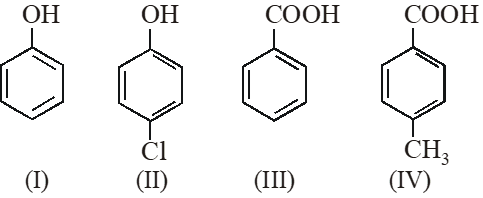(A) (III) > (IV) > (II) > (I) (B) (IV) > (III) > (I) > (II) (C) (III) > (II) > (I) > (IV) (D) (II) > (III) > (IV) > (I) [IIT-JEE-2009]
Ans. (A) (A) Acidity $\propto-R /-I \propto \frac{1}{+R /+I}$
Q. The correct stability order of the following resonance structures is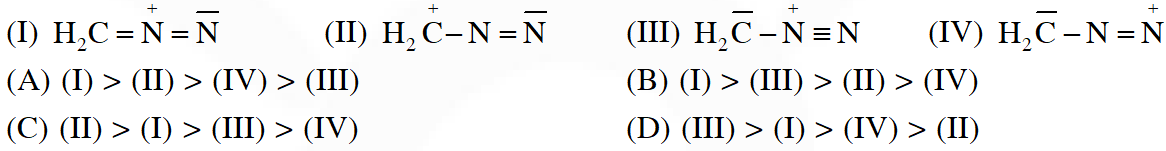[IIT-2009]
Ans. (B)
Q. Amongst the following, the total number of compounds soluble in aquesous NaOH is: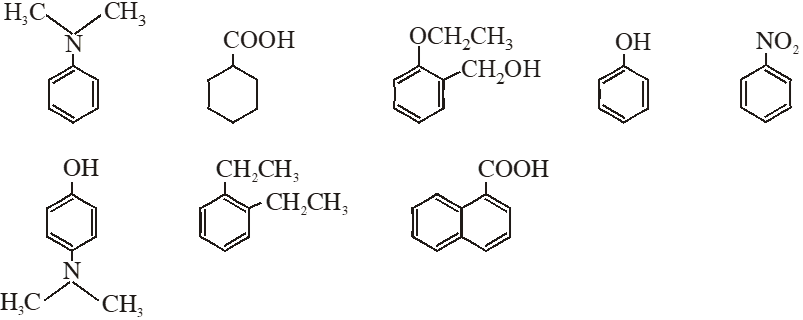[IIT-JEE-2010]
Ans. 4 (4) Compound which is more acidic than water is soluble in NaOH
Q. The total number of contributing structures showing hyperconjugation (involving C–H bonds) for the following carbocation is.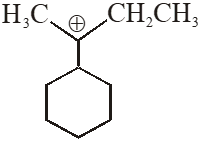[IIT-2011]
Ans. 6 $6 \propto \mathrm{H}$ ore present.
Q. Among the following compounds, the most acidic is – (A) p-nitrophenol (B) p-hydroxybenzoic acid (C) o-hydroxybenzoic acid (D) p-toluic acid [IIT-JEE-2011]
Ans. (C)
Q. Which of the following molecules, in pure from , is (are) unstable at room temperature ?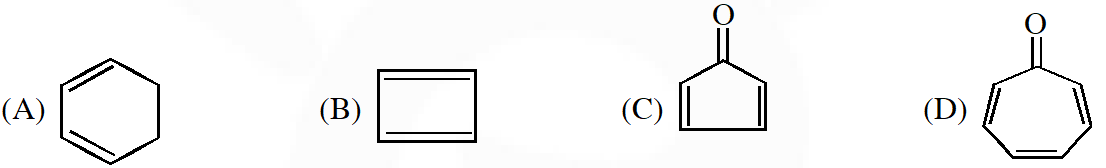[IIT-2012]
Ans. (B,C) Anti - Aromatic : B,C
Q. The carboxyl functional group (–COOH) is present in – (A) picric acid (B) barbituric acid (C) ascorbic acid (D) aspirin [IIT-JEE-2012]
Ans. (D)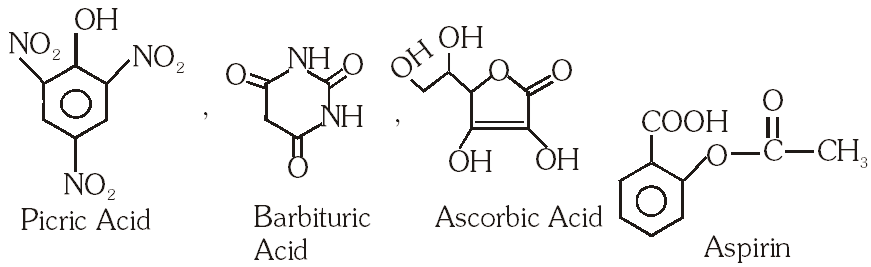Q. Identify the binary mixtures (s) that can be separated into the individual compounds, by differential extraction, as shown in the given scheme -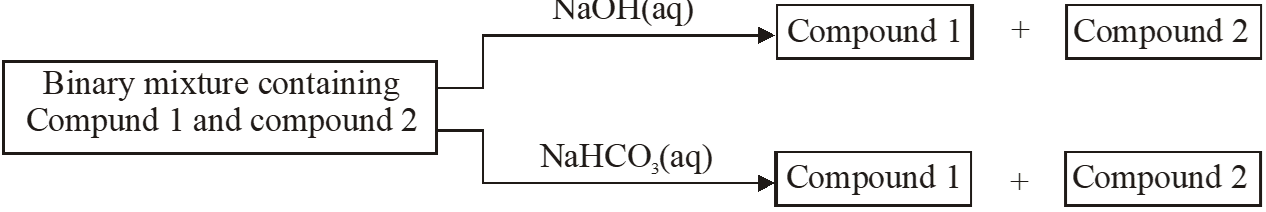(A) $\mathrm{C}_{6} \mathrm{H}_{5} \mathrm{OH}$ and $\mathrm{C}_{6} \mathrm{H}_{5} \mathrm{COOH}$ (B) $\mathrm{C}_{6} \mathrm{H}_{5} \mathrm{COOH}$ and $\mathrm{C}_{6} \mathrm{H}_{5} \mathrm{CH}_{2} \mathrm{OH}$ (C) $\mathrm{C}_{6} \mathrm{H}_{5} \mathrm{CH}_{2} \mathrm{OH}$ and $\mathrm{C}_{6} \mathrm{H}_{5} \mathrm{OH}$ (D) $\mathrm{C}_{6} \mathrm{H}_{5} \mathrm{CH}_{2} \mathrm{OH}$ and $\mathrm{C}_{6} \mathrm{H}_{5} \mathrm{CH}_{2} \mathrm{COOH}$ [IIT-JEE-2012]
Ans. (B,D)
Q. The hyperconjugative stbilities of tert-butyl cation and 2-butene, respectively, are due to – (A) $\sigma \rightarrow \mathrm{p}$ (empty) and $\sigma \rightarrow \pi$ electron delocalisations (B) $\sigma \rightarrow \sigma$ and $\sigma \rightarrow \pi$ electron delocalisations (C) $\sigma \rightarrow \mathrm{p}(\text { filled })$ and $\sigma \rightarrow \pi$ electron delocalisations (D) $\mathrm{p}$ (filled) $\rightarrow \sigma$ and $\sigma \rightarrow \pi$ electron delocalisations [IIT-2013]
Ans. (A)
Q. The total number of lone-pairs of electrons in melamine is - [IIT-2013]
Ans. 6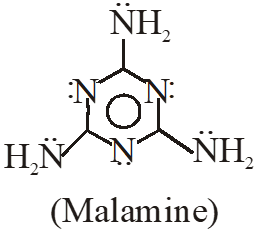Q. The compound that does NOT liberate $\mathrm{CO}_{2}$, on treatment with aqueous sodium bicarbonate solution, is – (A) Benzoic acid (B) Benzenesulphonic acid (C) Salicylic acid (D) Carbolic acid (phenol) [JEE-ADVANCED-2013]
Ans. (D) (D) Compound which is more acidic than $\mathrm{H}_{2} \mathrm{CO}_{3}$ liberate $\mathrm{CO}_{2}$ gas.
Q. Hydrogen bonding plays a central role in the following phenomena (A) Ice floats in water (B) Higher Lewis basicity of primary amines than tertiary amines in aqueous solutions (C) Formic acid is more acidic than acetic acid (D) Dimerisation of acetic acid in benzene [JEE-ADVANCED-2014]
Ans. (A,B,D)
Q. The number of resonance structures for N is :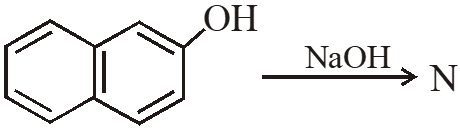[IIT-2015]
Ans. 9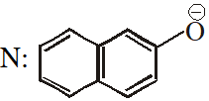VincentMum
Feb. 8, 2023, 1:32 p.m.
Thanks a lot, Quite a lot of write ups. https://ouressays.com/ mba dissertation writing
Error
Sept. 19, 2022, 3 p.m.
solution k liye app kyu download kree koi mtlb Banta hai free krooo bahot revolution la rhe ho toh
Nixson Nyonyi
March 25, 2022, 2:08 p.m.
F.B.I
Sept. 14, 2021, 7:36 p.m.
Well thanks
Lavanya
Sept. 26, 2020, 7:20 p.m.
Thanks
chandana Nayak
Sept. 25, 2020, 3:09 p.m.
chandana Nayak
Sept. 25, 2020, 3:08 p.m.
good work but some solutions are wrong
Debabrata Sahu
Sept. 18, 2020, 10 p.m.
Thanks a lot.
Rashika Gangwar
Sept. 17, 2020, 12:09 p.m.
Esaral is really helpful me a lot ...thanks
Sai Sri Ram Varun
Sept. 4, 2020, 8:05 p.m.
Good website helps people a lot 👍👍
Devil
Aug. 25, 2020, 11:33 a.m.
Solutions are not precise and some don't have any explaination.
Yuvraj prajapati
July 21, 2020, 7:12 p.m.
Nice questions and cake samay me NEET k liye hi achah bn payega
Samiran Dilipkumar Kulkarni
July 21, 2020, 11:08 a.m.
Good 😀😀
manik aggarwal
July 10, 2020, 4:55 p.m.
thanku
Priyanka naik
May 24, 2020, 11:41 p.m.
So nice and amazing. Question it's very useful for competitive exams
Priyanka nayak
May 24, 2020, 11:40 p.m.
So nice and amazing. Question it's very useful for competitive exams